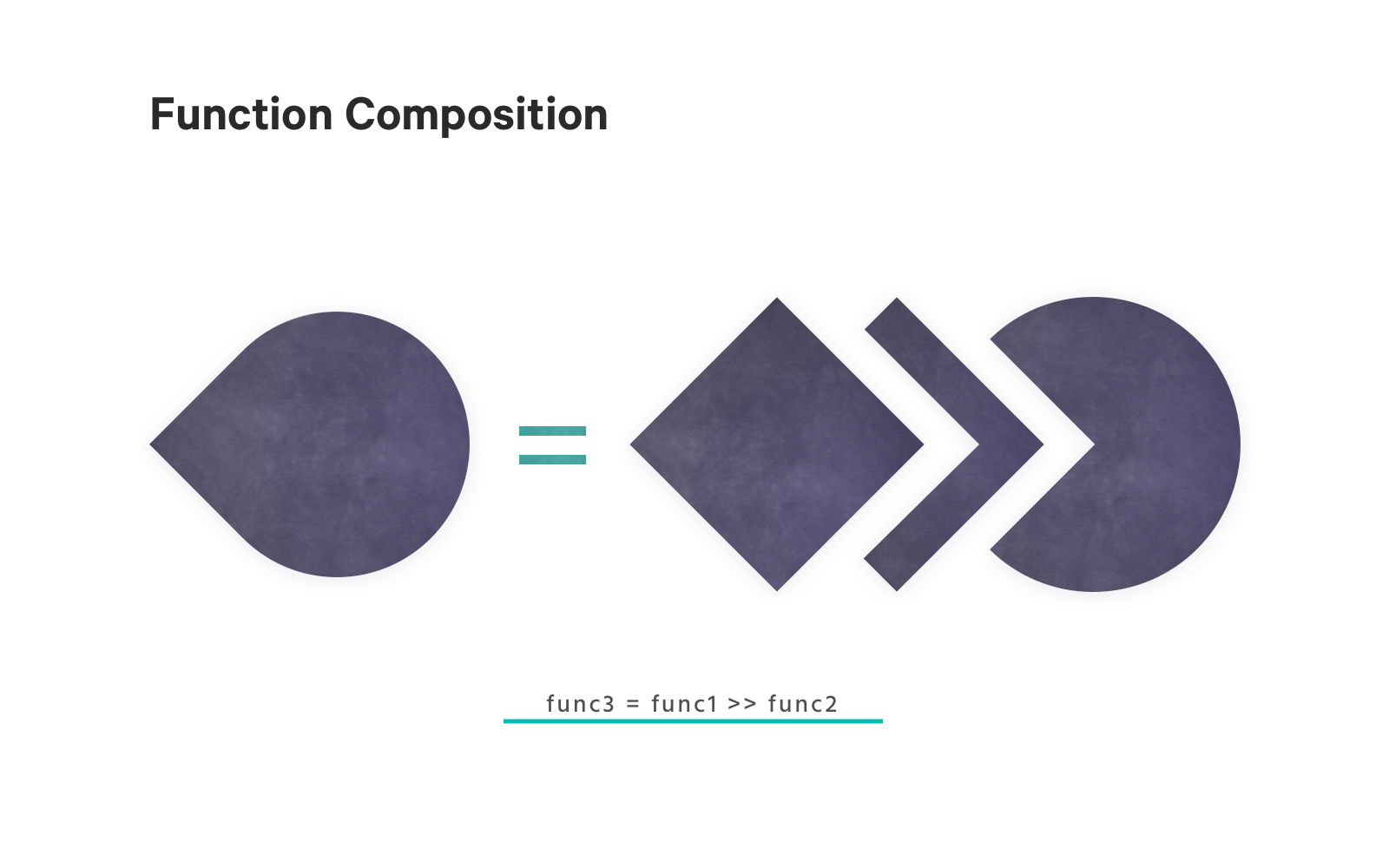# Function Composition in Ruby

Along with a number of other cool new features and performance improvements, Ruby 2.6 added function composition to the Proc and Method classes. Today we’ll take a look at how this allows us to use some functional programming goodness in our Ruby code.

# What is function composition?

So what is function composition? Simply put, function composition is the act of combining existing functions to create new functions. It’s a common technique in functional programming languages like Haskell and Elm.I’ll borrow some syntax from Haskell to describe this in a more concrete way (don’t worry, we’ll get to some Ruby shortly!) Let’s say we have two functions: `func1` and `func2`. The type signature for `func1` is:

``````func1 :: A -> B
``````

This means `func1` takes a value of type `A` and returns a value of type `B`. The type signature for `func2` is:

``````func2 :: B -> C
``````

So `func2` takes a value of type `B` and returns a value of type `C`. Using function composition, we can combine these two functions, creating a new function `func3` which takes a value of type `A` and returns a value of type `C`:

``````func3 :: A -> C
``````

This works because the type of value returned by `func1` matches the type of value `func2` expects as an argument. So our original value goes into `func1`, the value returned is then passed to `func2`, which then returns our final value.

# Let’s write some Ruby!

So how does function composition look in Ruby? Let’s look at a real(-ish) world example using Ruby `Proc`s. Let’s say we have defined the following:

``````BENEFIT_COST_IN_CENTS = 1000 * 100
TAX_RATE = 0.4

subtract_benefit = ->(salary) { salary - BENEFIT_COST_IN_CENTS }

pay_tax = ->(salary) { salary * (1 - TAX_RATE) }
``````

Invoking `subtract_benefit` subtracts the cost of a benefit from a salary:

``````SALARY_IN_CENTS = 15_000 * 100

subtract_benefit.call(SALARY_IN_CENTS) # => 1400000
``````

Invoking `pay_tax` subtracts the tax as a percentage from the salary:

``````pay_tax.call(SALARY_IN_CENTS) # => 900000.0
``````

Now we can compose these two with `Proc#>>` to create a new `Proc` which, given a salary, calculates our take home pay after tax and benefits:

``````calculate_take_home_pay = subtract_benefit >> pay_tax

calculate_take_home_pay.call(SALARY_IN_CENTS) # => 840000.0
``````

This is the same result we’d get if we nested then calls:

``````pay_tax.call(subtract_benefit.call(SALARY_IN_CENTS)) # => 840000.0
``````

As the employee, we’ve worked this to our advantage by subtracting the benefit amount before taking off the tax. If we pay tax and then subtract the benefit cost we’ll end up with a different result:

``````less_beneficial_calculate_take_home_pay = pay_tax >> subtract_benefit

less_beneficial_calculate_take_home_pay.call(SALARY_IN_CENTS) # => 800000.0 😞
``````

In this case we changed the order by reversing the order of the procs. We can also control the ordering with the operator we use. `Proc#>>` goes from left to right but its counterpart `Proc#<<` goes from right to left.

Let’s wrap up the example by defining a `Proc` which formats the salary nicely:

``````format_salary = ->(salary) { "\$#{format('%0.2f', salary / 100.0)}" }
``````

Now we combine this with `calculate_take_home_pay` to create a `Proc` which takes a salary and returns a nicely formatted take home amount:

``````formatted_take_home_pay = calculate_take_home_pay >> format_salary
formatted_take_home_pay.call(SALARY_IN_CENTS) # => "\$8400.00"
``````

# Pitfalls to Look Out For

When combining functions, we need to make sure that we’re composing in such a way that the types match. In our intro we borrowed some type signature examples from Haskell, which is statically typed. The Haskell compiler will let us know if we’re trying to compose functions whose input and output types are not cohesive, i.e. whose types don’t match.

Ruby is a little bit more flexible, and we rely on duck typing rather than on a static type system. But we can still combine functions in ways which don’t make sense, resulting in runtime errors.

Here we define two `Proc`s, `string_length` which takes a string and returns its length, and `exclaim` which takes a string and capitalizes and adds a “!” to the end:

``````string_length = ->(string) { string.length }
exclaim = ->(string) { "#{string.upcase}!" }
``````

Now we combine them into a function (of dubious usefulness) which takes a string, exclaims it, and returns the length:

``````exclaimed_length = exclaim >> length
exclaimed_length.call("ruby") # => 5
``````

So far so good. Now let’s see what happens if we reverse the order of composition:

``````exclaimed_length = length >> exclaim
exclaimed_length.call("ruby") # => undefined method `upcase' for 4:Integer
``````

Oops! We calculated the length first, which gave us `4`. But then we tried to call `#upcase` on the result, which doesn’t make sense because `Integer` doesn’t implement `#upcase`. In this situation I often think of the analogy of trying to fit together two mis-matching jigsaw puzzle pieces.

# Wrapping Up

One of the things I find interesting about programming languages is when ideas from one language influence the way you write and think about other languages, particularly those which use a different programming paradigm. So it’s great to see concepts from functional programming languages, like function composition, make it into an object-oriented language like Ruby.

I don’t think it’s the right tool for every job, and as programmers we have a responsibility to write code which is as clear and maintainable as possible. But being able to succinctly combine discreet functions into more complex, powerful functions is a tool I’m glad to have available.